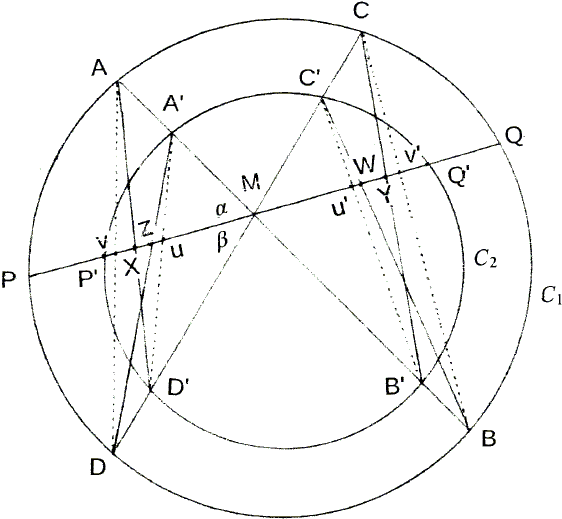# Butterflies in Similar Co-axial Conics

### Sidney Kung May 14, 2012Let there be two co-axial similar conic sections $C_1$ and $C_2$. A line crosses them at $P$, $P'$ and $Q$, $Q'$, $M$ being a point of $PQ$ and $P'Q'.$ Through $M$, draw two lines $AA'BB'$ and $CC'DD'$ and connect $AD',$ $A'D,$ $A'D',$ $AD,$ $BC',$ $B'C,$ $B'C',$ and $BC.$ Let $X,$ $Z,$ $U,$ $V,$ $Y,$ $W,$ $U',$ $V'$ be the points of intersection of $PP'Q'Q$ with the eight line segments, respectively. Then

 (1) $\frac{1}{MX} + \frac{1}{MZ} - \frac{1}{MP'} - \frac{1}{MP}= \frac{1}{MY} + \frac{1}{MW} - \frac{1}{MQ'} - \frac{1}{MQ}$.

(For convenience, we use the figure from the Better Butterfly ttheorem, so that in the diagram the two circles represent similar conics.)

For a proof, we apply the lemma from the Better Butterfly theorem page to triangles $MA'D',$ $MAD,$ $MA'D,$ and $MAD'$:

 (2) $\frac{\text{sin}(\alpha )}{MD'} + \frac{\text{sin}(\beta )}{MA'} = \frac{\text{sin}(\alpha +\beta )}{MU}$ $\frac{\text{sin}(\alpha )}{MD} + \frac{\text{sin}(\beta )}{MA} = \frac{\text{sin}(\alpha +\beta )}{MV}$ $\frac{\text{sin}(\alpha )}{MD} + \frac{\text{sin}(\beta )}{MA'} = \frac{\text{sin}(\alpha +\beta )}{MZ}$ $\frac{\text{sin}(\alpha )}{MD'} + \frac{\text{sin}(\beta )}{MA} = \frac{\text{sin}(\alpha +\beta )}{MX}$.

So

\begin{align} \frac{\text{sin}(\alpha +\beta )}{MU} + \frac{\text{sin}(\alpha +\beta )}{MV} &= \left(\frac{\text{sin}(\alpha )}{MD'} + \frac{\text{sin}(\beta )}{MA'}\right) + \left(\frac{\text{sin}(\alpha )}{MD} + \frac{\text{sin}(\beta )}{MA}\right) \\ &= \left(\frac{\text{sin}(\alpha )}{MD'} + \frac{\text{sin}(\beta )}{MA}\right) + \left(\frac{\text{sin}(\alpha )}{MD} + \frac{\text{sin}(\beta )}{MA'}\right) \\ &= \frac{\text{sin}(\alpha +\beta )}{MX} + \frac{\text{sin}(\alpha +\beta )}{MZ}. \end{align}

Hence,

 (3) $\frac{1}{MU} + \frac{1}{MV} = \frac{1}{MX} + \frac{1}{MZ}$.

Similarly,

 (4) $\frac{1}{MU'} + \frac{1}{MV'} = \frac{1}{MW} + \frac{1}{MY}$.

Now, consider the inner circle as a conic section to which we apply the result of E. J. Atzema:

 (5) $\frac{1}{MU} - \frac{1}{MP'} = \frac{1}{MU'} - \frac{1}{MQ'}$.

Likewise, for the outer circle, we have

 (6) $\frac{1}{MV} - \frac{1}{MP} = \frac{1}{MV'} - \frac{1}{MQ}$.

Adding (5) and (6) and taking into account (3) and( 4), we get (1).### Butterfly Theorem and Variants